# Intro

The grid package is what makes R graphics great. All the popular tools with awesome graphics use the grid as the backend, e.g. ggplot2 and lattice. When I started working on the forestplot package I first encountered the grid and it was instant love. In this vignette I’ll show how you can use the box-functions in this package together with grid in order to generate a flowchart.

# Basics

The grid package splits the plot into views. You can define a viewport and it will work as an isolated part of the plot, ignorant of the world around it. You do this via viewport, below I create a plot and add a rectangle to it:

# Load the grid library
# part of standard R libraries so no need installing
library(grid)

# Create a new graph
grid.newpage()

pushViewport(viewport(width=.5, height=.8))

grid.rect(gp=gpar(fill="#D8F0D1"))

popViewport()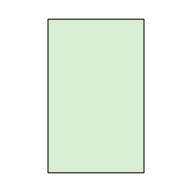Important to note is that the grid allows you to define precise units or relative units.

## Relative units

• npc - ranges from 0-1 where 1 is 100% of the viewport width.
• snpc - similar to npc but is the same length in height/width.
• lines - the height of a line. The go-to method if you want to know the height of a few lines of text. It’s relative to the viewport’s fontsize and lineheight.
• char - the lines without the lineheight part.

Below we draw a line with relative units in two nested viewports. Note that the to lines are generated from the exact same grob object but appear different depending on the viewport they are in:

grid.newpage()
pushViewport(viewport(width=.5, height=.8, clip="on"))
grid.rect(gp=gpar(lty=2, fill="lightyellow"))
lg <- linesGrob(x = unit(c(.2, 1), "npc"),
y = unit(c(.2, 1), "npc"),
gp = gpar(lwd=2))
grid.draw(lg)
pushViewport(viewport(x=0, y=.6, just="left", width=.4, height=.4, angle = 20))
grid.rect(gp=gpar(fill="lightblue")) # A translucent box to indicate the new viewport
grid.draw(lg)
popViewport()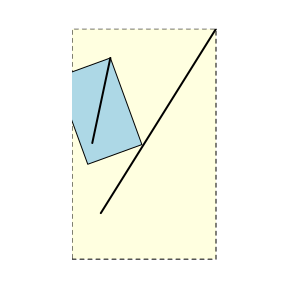## Absolute units

• mm - probably my go-to unit when I want something absolute.
• inch - if you prefer inches I guess this is the go-to choice.

Below we draw a line with absolute units in two nested viewports. Note that the lines have the exact same length:

grid.newpage()
pushViewport(viewport(width=.5, height=.8, clip="on"))
grid.rect(gp=gpar(lty=2, fill="lightyellow"))
lg <- linesGrob(x = unit(c(2, 10), "mm"),
y = unit(c(2, 10), "mm"),
gp = gpar(lwd=2))
grid.draw(lg)
pushViewport(viewport(x=0, y=.6, just="left", width=.4, height=.4, angle = 20))
grid.rect(gp=gpar(fill="lightblue")) # A translucent box to indicate the new viewport
grid.draw(lg)
popViewport()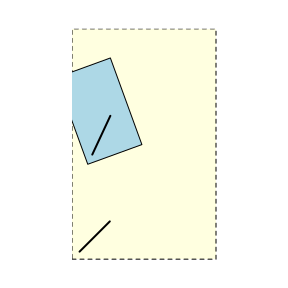# A basic box

Now we can start exploring how to use the Gmics::box functions. We’ll strt with outputting a single box:

library(Gmisc)
grid.newpage()
txt <-
"Just a plain box
with some text
- Note that newline is OK"
boxGrob(txt)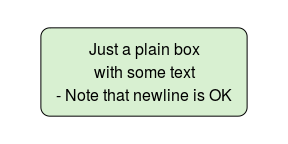We can position and style this box as any element:

grid.newpage()
boxGrob("A large\noffset\nyellow\nbox",
width=.8, height=.8,
x=0, y=0,
bjust = c(0,0),
txt_gp = gpar(col="darkblue", cex=2),
box_gp = gpar(fill="lightyellow", col="darkblue"))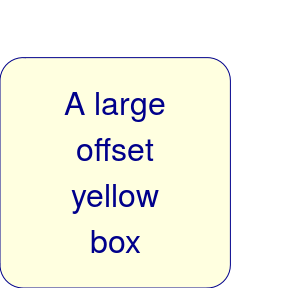# A box with proportiions

The boxPropGrob is for displaying proportions as the name indicates.

grid.newpage()
boxPropGrob("A box with proportions",
"Left side", "Right side",
prop=.7)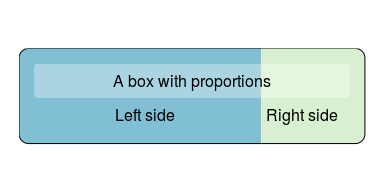# The box coordinates

The boxes have coordinates that allow you to easily draw lines to and from it. The coordinates are stored in the coords attribute. Below is an illustration of the coordinates for the two boxes:

grid.newpage()
smpl_bx <- boxGrob(
label = "A simple box",
x = .5,
y = .9,
just = "center")

prop_bx <- boxPropGrob(
label = "A split box",
label_left = "Left side",
label_right = "Right side",
x = .5,
y = .3,
prop = .3,
just = "center")

plot(smpl_bx)
plot(prop_bx)

smpl_bx_coords <- attr(smpl_bx, "coords")
grid.circle(y = smpl_bx_coords$y, x= smpl_bx_coords$x, r = unit(2, "mm"), gp=gpar(fill="#FFFFFF99", col="black"))
grid.circle(y = smpl_bx_coords$bottom, x= smpl_bx_coords$right, r = unit(1, "mm"), gp=gpar(fill="red"))
grid.circle(y = smpl_bx_coords$top, x = smpl_bx_coords$right, r = unit(1, "mm"), gp=gpar(fill="purple"))
grid.circle(y = smpl_bx_coords$bottom, x = smpl_bx_coords$left, r = unit(1, "mm"), gp=gpar(fill="blue"))
grid.circle(y = smpl_bx_coords$top, x = smpl_bx_coords$left, r = unit(1, "mm"), gp=gpar(fill="orange"))

prop_bx_coords <- attr(prop_bx, "coords")
grid.circle(y = prop_bx_coords$y, x= prop_bx_coords$x, r = unit(2, "mm"), gp=gpar(fill="#FFFFFF99", col="black"))
grid.circle(y = prop_bx_coords$bottom, x= prop_bx_coords$right_x, r = unit(1, "mm"), gp=gpar(fill="red"))
grid.circle(y = prop_bx_coords$top, x = prop_bx_coords$right_x, r = unit(1, "mm"), gp=gpar(fill="purple"))
grid.circle(y = prop_bx_coords$bottom, x = prop_bx_coords$left_x, r = unit(1, "mm"), gp=gpar(fill="blue"))
grid.circle(y = prop_bx_coords$top, x = prop_bx_coords$left_x, r = unit(1, "mm"), gp=gpar(fill="orange"))

grid.circle(y = prop_bx_coords$bottom, x= prop_bx_coords$right, r = unit(2, "mm"), gp=gpar(fill="red"))
grid.circle(y = prop_bx_coords$top, x = prop_bx_coords$right, r = unit(2, "mm"), gp=gpar(fill="purple"))
grid.circle(y = prop_bx_coords$bottom, x = prop_bx_coords$left, r = unit(2, "mm"), gp=gpar(fill="blue"))
grid.circle(y = prop_bx_coords$top, x = prop_bx_coords$left, r = unit(2, "mm"), gp=gpar(fill="orange"))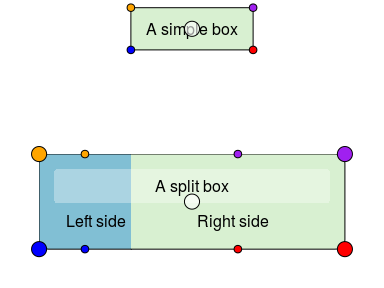# Connecting the boxes

In order to make connecting boxes with an arrow there is the connectGrob function. Here’s an example of how you can use it for connecting a set of boxes:

grid.newpage()

# Initiate the boxes that we want to connect
side <- boxPropGrob("Side", "Left", "Right",
prop=.3,
x=0, y=.9,
bjust = c(0,1))
start <- boxGrob("Top",
x=.6, y=coords(side)$y, box_gp = gpar(fill = "yellow")) bottom <- boxGrob("Bottom", x=.6, y=0, bjust="bottom") sub_side_left <- boxGrob("Left", x = coords(side)$left_x,
y = 0,
bjust = "bottom")
sub_side_right <- boxGrob("Right",
x = coords(side)$right_x, y = 0, bjust = "bottom") odd <- boxGrob("Odd\nbox", x=coords(side)$right,
y=.5)

odd2 <- boxGrob("Also odd",
x=coords(odd)$right + distance(bottom, odd, type="h", half=TRUE) - unit(2, "mm"), y=0, bjust = c(1,0)) exclude <- boxGrob("Exclude:\n - Too sick\n - Prev. surgery", x=1, y=coords(bottom)$top +
distance(start, bottom,
type="v", half=TRUE),
just="left", bjust = "right")

# Connect the boxes and print/plot them
connectGrob(start, bottom, "vertical")
connectGrob(start, side, "horizontal")
connectGrob(bottom, odd, "Z", "l")
connectGrob(odd, odd2, "N", "l")
connectGrob(side, sub_side_left, "v", "l")
connectGrob(side, sub_side_right, "v", "r")
connectGrob(start, exclude, "-",
lty_gp = gpar(lwd=2, col="darkred", fill="darkred"))

# Print the grobs
start
bottom
side
exclude
sub_side_left
sub_side_right
odd
odd2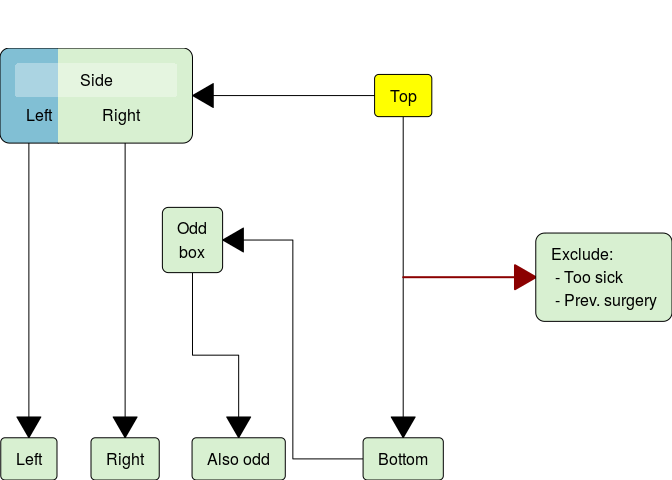# Expressions in boxes

It is possible to use the R expression function to produce bold or italics text, or even formulae.

A few pointers on expression

• expressions with multiple elements should be combined using paste. E.g. expression(paste(beta, "1")) would produce $$\beta1$$
• the behaviour of paste when used in expression is more like the normal behaviour or paste0 (i.e. no separating space)
• greek letters can be entered outside of quotes by typing the name e.g. expression(beta) will become $$\beta$$ and expression(Gamma) will become $$\Gamma$$ (note the case, not all greek letters are available in upper case)
• superscripts are done via expression(x^2) and subscripts via expression(x)
grid.newpage()
boxGrob(expression(bold("Bold text")), 0.8, 0.3)
boxGrob(expression(italic("Italics text")), 0.8, 0.7)
boxGrob(expression(paste("Mixed: ", italic("Italics"), " and ", bold("bold"))), 0.6, 0.5)
boxGrob(expression(paste("y = ", beta, " + ", beta, X, " + ", beta, X^2)), 0.35, 0.5)
boxGrob(expression(paste(beta, gamma, Gamma)), 0.15, 0.5)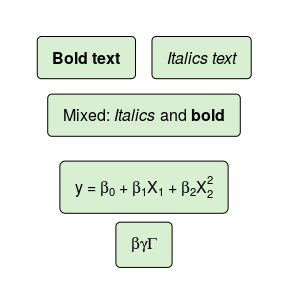See the plotmath helpfile for more details.

# Tips for debugging

If you find that your elements don’t look as expected make sure that your not changing viewport/device. While most coordinates are relative some of them need to be fixed and therefore changing the viewport may impact where elements are renered.